## 4. Interference.

The mechanical behavior of point P of a continuous elastic medium where overlap two different waves Ψ1 e Ψ2, emitted by two sources O1 e O2 from which it has distances r1 e r2, is the same as if there were only one wave given by the sum of Ψ1 e Ψ2.

In particular, if the sources O1 e O2 oscillate in phase with equal amplitudes and angular frequencies, the prosthaphaeresis formulas give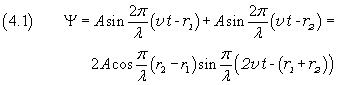The amplitude of the sum Ψ is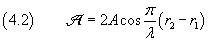This amplitude has its maximum value 2A when the absolute value of the cosine in (4.2) is 1, that is when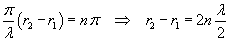The points of the medium such that the difference between their distances from the sources O1 and O2 is equal to an even number of half-wavelengths oscillate with the greatest amplitude.

The resulting amplitude instead is null, that is the point P doesn't oscillate, when the cosine is null, that is when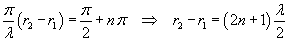The points of the medium such that the difference between their distances from the sources O1 and O2 is equal to an odd number of half-wavelengths do not oscillate.

In conclusion, when there are two sources with equal amplitudes and angular frequencies, the points of a medium oscillate with different amplitudes, from a minimum value 0 to a maximum value 2A. In a three-dimensional medium the sets of contiguous points which do not oscillate are hyperboloidal nodal surfaces and the sets of contiguous points which oscillate with greatest amplitude are hyperboloidal antinodal surfaces.

In particular, in a one-dimensional medium, these surfaces reduce to points said respectively nodes and antinodes. In this case, the intermediate point between two sources is an antinode.

You can see some maybe useful animations in Waves.

If instead the sources O1 e O2 are in antiphase, that is they have phase difference π or, more simply, they oscillate like the pans of a balance, the wavefunctions are, with an appropriate choice of the origin of the time,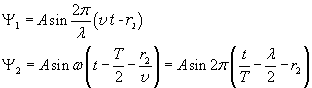The situation is the same as the sources were oscillating in phase, but at a distance from each other increased by an half-wavelength. Now the points of the medium are nodes when the difference between their distances from the sources O1 and O2 is equal to an even number of half-wavelengths and vice versa.

In particular, the intermediate point between two sources is a node.